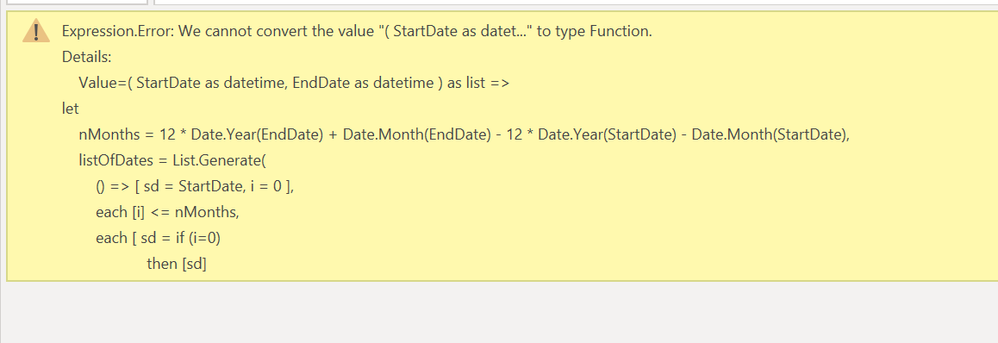cancel
Showing results for
Did you mean:Helper I

## power query loop or splite period to each month

Hi,

I have a table with fields "start date", "end date" and some other fields. For each record that "start date" and "end date" are not in the same month, I want to splite them to each month. For example, startdate is Jan 16th and enddate is Mar 13th, I want to have three records, one is Jan 16th to Jan 31st, the second one is Feb 1st to Feb 28th and the third one is Mar 1st to Mar 13th. Other fields just copying. How can I get it?

Thanks.

1 ACCEPTED SOLUTIONResolver IV

Good day yishenhui

To achieve the result you are looking for I first created a custom function. It takes Start Date and End Date as parameters.

It generates a list of dates. The first date in the list is Start Date. Any subsequent dates are the first day of each month. The last date in the list is the first day of the month End Date falls within.

I invoke the function to create a new column of lists. This column is called Start Dates Split. I expand the column.

Finally I calculate the end date for each month. This is calculated as the lesser of the End Date and the end of the month of Start Dates Split.

Here is my M code for the custom function

`( StartDate as date, EndDate as date ) as list =>let    nMonths = 12 * Date.Year(EndDate) + Date.Month(EndDate) - 12 * Date.Year(StartDate) - Date.Month(StartDate),    listOfDates = List.Generate(        () => [ sd = StartDate, i = 0 ],        each [i] <= nMonths,        each [ sd = if (i=0)                    then [sd]                    else Date.StartOfMonth( Date.AddMonths( StartDate, i ) ),               i= [i] + 1        ],        each [sd]    )in    listOfDates`

Here is my M code for transforming the table

`let    Source = Excel.CurrentWorkbook(){[Name="Table1"]}[Content],    #"Changed Type" = Table.TransformColumnTypes(Source,{{"Start Date", type date}, {"End Date", type date}, {"ID", type text}, {"Comment", type text}}),    #"List of Start Dates" = Table.AddColumn(#"Changed Type",         "Start Dates Split",         each #"Generate Start Dates"([Start Date], [End Date])    ),    #"Expand List" = Table.ExpandListColumn(#"List of Start Dates", "Start Dates Split"),    #"Changed Type2" = Table.TransformColumnTypes(#"Expand List",{{"Start Dates Split", type date}}),    #"Add End Dates" = Table.AddColumn(#"Changed Type2",         "End Dates Split",         each List.Min( {Date.EndOfMonth( [Start Dates Split] ), [End Date] }),         type date    )in    #"Add End Dates"`

Here is my data (at step "Changed Type").Here is my result3 REPLIES 3Resolver IV

Good day yishenhui

To achieve the result you are looking for I first created a custom function. It takes Start Date and End Date as parameters.

It generates a list of dates. The first date in the list is Start Date. Any subsequent dates are the first day of each month. The last date in the list is the first day of the month End Date falls within.

I invoke the function to create a new column of lists. This column is called Start Dates Split. I expand the column.

Finally I calculate the end date for each month. This is calculated as the lesser of the End Date and the end of the month of Start Dates Split.

Here is my M code for the custom function

`( StartDate as date, EndDate as date ) as list =>let    nMonths = 12 * Date.Year(EndDate) + Date.Month(EndDate) - 12 * Date.Year(StartDate) - Date.Month(StartDate),    listOfDates = List.Generate(        () => [ sd = StartDate, i = 0 ],        each [i] <= nMonths,        each [ sd = if (i=0)                    then [sd]                    else Date.StartOfMonth( Date.AddMonths( StartDate, i ) ),               i= [i] + 1        ],        each [sd]    )in    listOfDates`

Here is my M code for transforming the table

`let    Source = Excel.CurrentWorkbook(){[Name="Table1"]}[Content],    #"Changed Type" = Table.TransformColumnTypes(Source,{{"Start Date", type date}, {"End Date", type date}, {"ID", type text}, {"Comment", type text}}),    #"List of Start Dates" = Table.AddColumn(#"Changed Type",         "Start Dates Split",         each #"Generate Start Dates"([Start Date], [End Date])    ),    #"Expand List" = Table.ExpandListColumn(#"List of Start Dates", "Start Dates Split"),    #"Changed Type2" = Table.TransformColumnTypes(#"Expand List",{{"Start Dates Split", type date}}),    #"Add End Dates" = Table.AddColumn(#"Changed Type2",         "End Dates Split",         each List.Min( {Date.EndOfMonth( [Start Dates Split] ), [End Date] }),         type date    )in    #"Add End Dates"`

Here is my data (at step "Changed Type").Here is my resultHelper I

Hi Collinsg,

Not sure if I did something wrong in create custom function. It's the first time I create custom function. What I did is like below (only change the type from date to datetime). in the M query, I added other M code (changed the type as well). But I got error msg when I invoke the function.Helper I

Hi Collinsg,

I was able to figure out the mistake I made. I will accept your reply as solution.

Thanks.